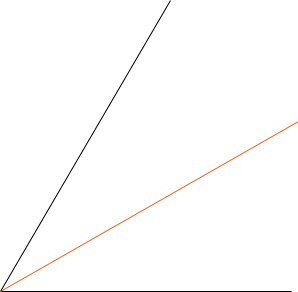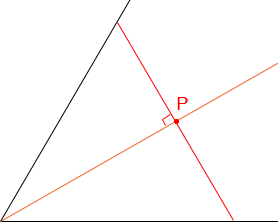SEARCH HOMEMath Central Quandaries & QueriesHello, I'm Christine. I'm doing an project in school about tessellations. But first, I have to construct an equilateral triangle with an altitude of exactly 2 inches. I know how to draw an equilateral triangle...but I don't know what I have to do in order for the triangle to have an altitude of 2 inches. Thank you, ChristineHi Christine,

By construct I expect that you mean with straight edge and compass but in addition you have a way to measure 2 inches.

I would start by constructing a 60 degree angle and then bisecting it.Now measure along the bisector a distance of 2 inches to a point P. At P construct a line which is perpendicular to the bisector.Now you have an equilateral triangle. Can you see why?

PennyMath Central is supported by the University of Regina and The Pacific Institute for the Mathematical Sciences.